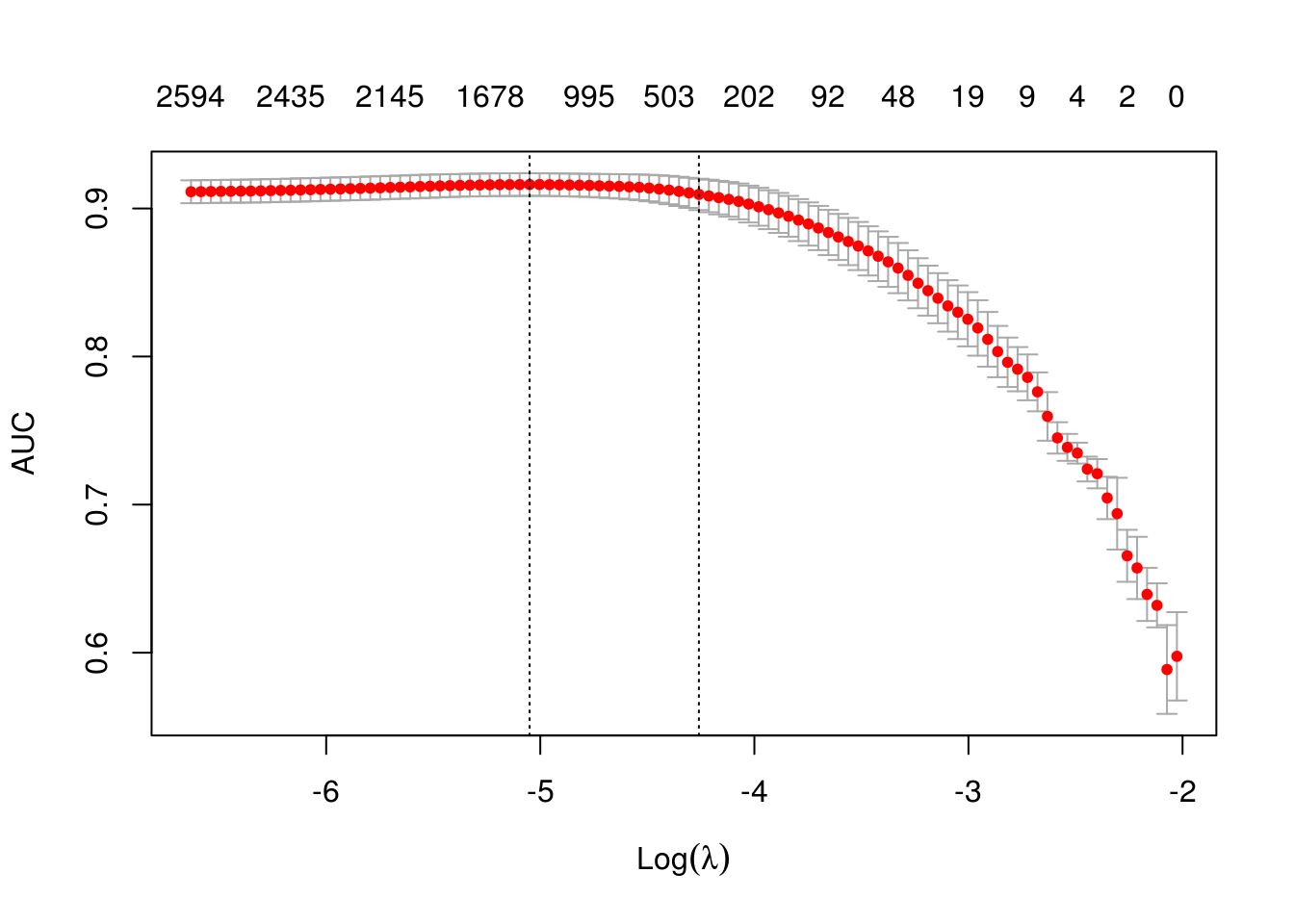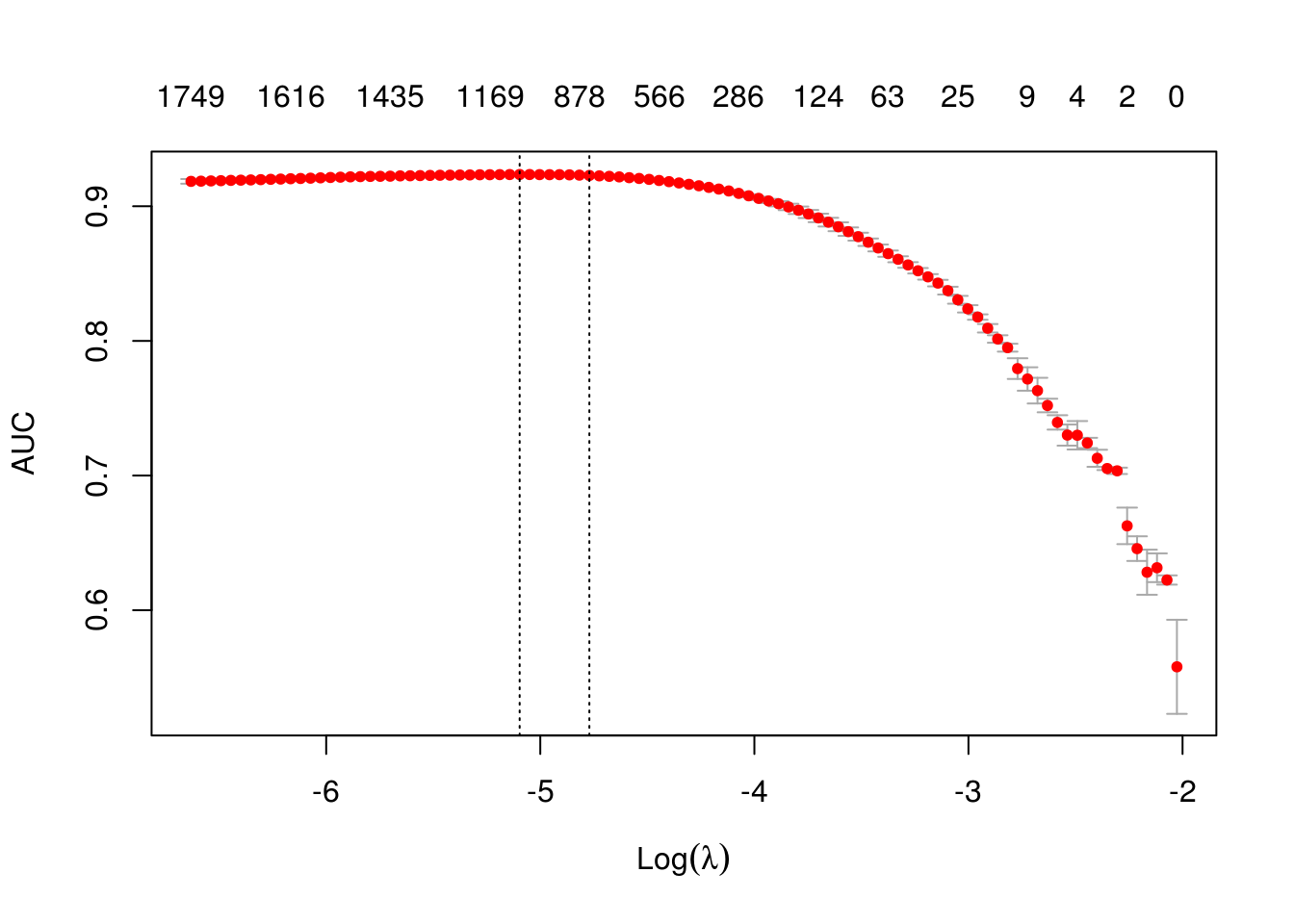# Text analysis pipeline

Most text mining and NLP modeling use bag of words or bag of n-grams methods. Despite their simplicity, these models usually demonstrate good performance on text categorization and classification tasks. But in contrast to their theoretical simplicity and practical efficiency building bag-of-words models involves technical challenges. This is especially the case in R because of its copy-on-modify semantics.

Let’s briefly review some of the steps in a typical text analysis pipeline:

1. The reseacher usually begins by constructing a document-term matrix (DTM) or term-co-occurence matrix (TCM) from input documents. In other words, the first step is to vectorize text by creating a map from words or n-grams to a vector space.
2. The researcher fits a model to that DTM. These models might include text classification, topic modeling, similarity search, etc. Fitting the model will include tuning and validating the model.
3. Finally the researcher applies the model to new data.

In this vignette we will primarily discuss the first step. Texts themselves can take up a lot of memory, but vectorized texts usually do not, because they are stored as sparse matrices. Because of R’s copy-on-modify semantics, it is not easy to iteratively grow a DTM. Thus constructing a DTM, even for a small collections of documents, can be a serious bottleneck for analysts and researchers. It involves reading the whole collection of text documents into RAM and processing it as single vector, which can easily increase memory use by a factor of 2 to 4. The text2vec package solves this problem by providing a better way of constructing a document-term matrix.

Let’s demonstrate package core functionality by applying it to a real case problem - sentiment analysis.

text2vec package provides the `movie_review` dataset. It consists of 5000 movie reviews, each of which is marked as positive or negative. We will also use the data.table package for data wrangling.

First of all let’s split out dataset into two parts - train and test. We will show how to perform data manipulations on train set and then apply exactly the same manipulations on the test set:

``````library(text2vec)
library(data.table)
library(magrittr)
data("movie_review")
setDT(movie_review)
setkey(movie_review, id)
set.seed(2017L)
all_ids = movie_review\$id
train_ids = sample(all_ids, 4000)
test_ids = setdiff(all_ids, train_ids)
train = movie_review[J(train_ids)]
test = movie_review[J(test_ids)]``````

# Vectorization

To represent documents in vector space, we first have to create mappings from terms to term IDS. We call them terms instead of words because they can be arbitrary n-grams not just single words. We represent a set of documents as a sparse matrix, where each row corresponds to a document and each column corresponds to a term. This can be done in 2 ways: using the vocabulary itself or by feature hashing.

## Vocabulary-based vectorization

Let’s first create a vocabulary-based DTM. Here we collect unique terms from all documents and mark each of them with a unique ID using the `create_vocabulary()` function. We use an iterator to create the vocabulary.

``````# define preprocessing function and tokenization function
prep_fun = tolower
tok_fun = word_tokenizer

it_train = itoken(train\$review,
preprocessor = prep_fun,
tokenizer = tok_fun,
ids = train\$id,
progressbar = FALSE)
vocab = create_vocabulary(it_train)``````

What was done here?

1. We created an iterator over tokens with the `itoken()` function. All functions prefixed with `create_` work with these iterators. R users might find this idiom unusual, but the iterator abstraction allows us to hide most of details about input and to process data in memory-friendly chunks.
2. We built the vocabulary with the `create_vocabulary()` function.

Alternatively, we could create list of tokens and reuse it in further steps. Each element of the list should represent a document, and each element should be a character vector of tokens.

``````train_tokens = tok_fun(prep_fun(train\$review))

it_train = itoken(train_tokens,
ids = train\$id,
# turn off progressbar because it won't look nice in rmd
progressbar = FALSE)

vocab = create_vocabulary(it_train)
vocab``````
``````## Number of docs: 4000
## 0 stopwords:  ...
## ngram_min = 1; ngram_max = 1
## Vocabulary:
##        term term_count doc_count
##     1: 0.02          1         1
##     2:  0.3          1         1
##     3: 0.48          1         1
##     4:  0.5          1         1
##     5: 0.89          1         1
##    ---
## 38450:   to      21891      3796
## 38451:   of      23477      3794
## 38452:    a      26398      3880
## 38453:  and      26917      3868
## 38454:  the      53871      3970``````

Note that text2vec provides a few tokenizer functions (see `?tokenizers`). These are just simple wrappers for the `base::gsub()` function and are not very fast or flexible. If you need something smarter or faster you can use the tokenizers package which will cover most use cases, or write your own tokenizer using the stringi package.

Now that we have a vocabulary, we can construct a document-term matrix.

``````vectorizer = vocab_vectorizer(vocab)
t1 = Sys.time()
dtm_train = create_dtm(it_train, vectorizer)
print(difftime(Sys.time(), t1, units = 'sec'))``````
``## Time difference of 0.5483496 secs``

Now we have a DTM and can check its dimensions.

``dim(dtm_train)``
``##   4000 38454``
``identical(rownames(dtm_train), train\$id)``
``##  TRUE``

As you can see, the DTM has 4000 rows, equal to the number of documents, and 38454 columns, equal to the number of unique terms.

Now we are ready to fit our first model. Here we will use the glmnet package to fit a logistic regression model with an L1 penalty and 4 fold cross-validation.

``````library(glmnet)
NFOLDS = 4
t1 = Sys.time()
glmnet_classifier = cv.glmnet(x = dtm_train, y = train[['sentiment']],
family = 'binomial',
# L1 penalty
alpha = 1,
# interested in the area under ROC curve
type.measure = "auc",
# 5-fold cross-validation
nfolds = NFOLDS,
# high value is less accurate, but has faster training
thresh = 1e-3,
# again lower number of iterations for faster training
maxit = 1e3)
print(difftime(Sys.time(), t1, units = 'sec'))``````
``## Time difference of 2.805214 secs``
``plot(glmnet_classifier)````print(paste("max AUC =", round(max(glmnet_classifier\$cvm), 4)))``
``##  "max AUC = 0.9162"``

We have successfully fit a model to our DTM. Now we can check the model’s performance on test data. Note that we use exactly the same functions from prepossessing and tokenization. Also we reuse/use the same `vectorizer` - function which maps terms to indices.

``````# Note that most text2vec functions are pipe friendly!
it_test = tok_fun(prep_fun(test\$review))
# turn off progressbar because it won't look nice in rmd
it_test = itoken(it_test, ids = test\$id, progressbar = FALSE)

dtm_test = create_dtm(it_test, vectorizer)

preds = predict(glmnet_classifier, dtm_test, type = 'response')[,1]
glmnet:::auc(test\$sentiment, preds)``````
``##  0.9164517``

As we can see, performance on the test data is roughly the same as we expect from cross-validation.

### Pruning vocabulary

We can note, however, that the training time for our model was quite high. We can reduce it and also significantly improve accuracy by pruning the vocabulary.

For example, we can find words “a”, “the”, “in”, “I”, “you”, “on”, etc in almost all documents, but they do not provide much useful information. Usually such words are called stop words. On the other hand, the corpus also contains very uncommon terms, which are contained in only a few documents. These terms are also useless, because we don’t have sufficient statistics for them. Here we will remove pre-defined stopwords, very common and very unusual terms.

``````stop_words = c("i", "me", "my", "myself", "we", "our", "ours", "ourselves", "you", "your", "yours")
t1 = Sys.time()
vocab = create_vocabulary(it_train, stopwords = stop_words)
print(difftime(Sys.time(), t1, units = 'sec'))``````
``## Time difference of 0.3008301 secs``
``````pruned_vocab = prune_vocabulary(vocab,
term_count_min = 10,
doc_proportion_max = 0.5,
doc_proportion_min = 0.001)
vectorizer = vocab_vectorizer(pruned_vocab)
# create dtm_train with new pruned vocabulary vectorizer
t1 = Sys.time()
dtm_train  = create_dtm(it_train, vectorizer)
print(difftime(Sys.time(), t1, units = 'sec'))``````
``## Time difference of 0.2928042 secs``
``dim(dtm_train)``
``##  4000 6542``

Note that the new DTM has many fewer columns than the original DTM. This usually leads to both accuracy improvement (because we removed “noise”) and reduction of the training time.

Also we need to create DTM for test data with the same vectorizer:

``````dtm_test = create_dtm(it_test, vectorizer)
dim(dtm_test)``````
``##  1000 6542``

## N-grams

Can we improve the model? Definitely - we can use n-grams instead of words. Here we will use up to 2-grams:

``````t1 = Sys.time()
vocab = create_vocabulary(it_train, ngram = c(1L, 2L))
print(difftime(Sys.time(), t1, units = 'sec'))``````
``## Time difference of 1.311055 secs``
``````vocab = prune_vocabulary(vocab, term_count_min = 10,
doc_proportion_max = 0.5)

bigram_vectorizer = vocab_vectorizer(vocab)

dtm_train = create_dtm(it_train, bigram_vectorizer)

t1 = Sys.time()
glmnet_classifier = cv.glmnet(x = dtm_train, y = train[['sentiment']],
family = 'binomial',
alpha = 1,
type.measure = "auc",
nfolds = NFOLDS,
thresh = 1e-3,
maxit = 1e3)
print(difftime(Sys.time(), t1, units = 'sec'))``````
``## Time difference of 2.535554 secs``
``plot(glmnet_classifier)````print(paste("max AUC =", round(max(glmnet_classifier\$cvm), 4)))``
``##  "max AUC = 0.9236"``

Seems that usage of n-grams improved our model a little bit more. Let’s check performance on test dataset:

``````# apply vectorizer
dtm_test = create_dtm(it_test, bigram_vectorizer)
preds = predict(glmnet_classifier, dtm_test, type = 'response')[,1]
glmnet:::auc(test\$sentiment, preds)``````
``##  0.9294806``

Further tuning is left up to the reader.

## Feature hashing

If you are not familiar with feature hashing (the so-called “hashing trick”) I recommend you start with the Wikipedia article, then read the original paper by a Yahoo! research team. This technique is very fast because we don’t have to perform a lookup over an associative array. Another benefit is that it leads to a very low memory footprint, since we can map an arbitrary number of features into much more compact space. This method was popularized by Yahoo! and is widely used in Vowpal Wabbit.

Here is how to use feature hashing in text2vec.

``````h_vectorizer = hash_vectorizer(hash_size = 2 ^ 14, ngram = c(1L, 2L))

t1 = Sys.time()
dtm_train = create_dtm(it_train, h_vectorizer)
print(difftime(Sys.time(), t1, units = 'sec'))``````
``## Time difference of 0.883918 secs``
``````t1 = Sys.time()
glmnet_classifier = cv.glmnet(x = dtm_train, y = train[['sentiment']],
family = 'binomial',
alpha = 1,
type.measure = "auc",
nfolds = 5,
thresh = 1e-3,
maxit = 1e3)
print(difftime(Sys.time(), t1, units = 'sec'))``````
``## Time difference of 4.401476 secs``
``plot(glmnet_classifier)``